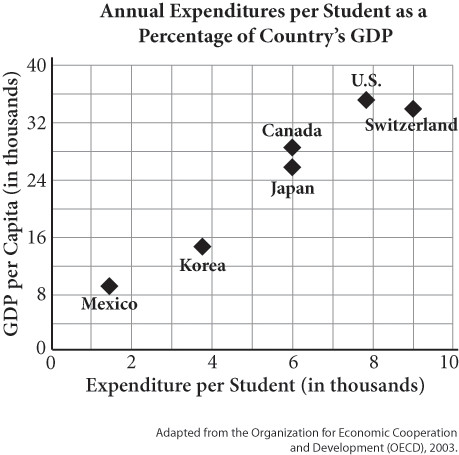# SAT Math Multiple Choice Question 559: Answer and Explanation

### Test Information

Question: 559

4.A student looked at the graph above and determined based on the data that spending more money per student causes the gross domestic product (GDP) to increase. Which of the following statements is true?

• A. The student is correct; the data shows that increased spending on students causes an increase in the GDP.
• B. The student is incorrect; the data shows that having a higher GDP causes an increase in the amount of money a country spends on students.
• C. The student is incorrect; there is no correlation and, therefore, no causation between GDP and expenditures on students.
• D. The student is incorrect; the two variables are correlated, but changes in one do not necessarily cause changes in the other.